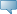# LCC

## Detailed Course Information

Spring 2012
Feb 25, 2020When the term course schedule is available the "Schedule Types" are hyperlinks. Clicking on the link will then display individual section information.

 MATH 126 - Precalculus Intensive course covering the same material as MATH 121-122. Topics include algebraic and transcendental functions, solving equations/inequalities algebraically and graphically, mathematical modeling, trigonometric identities, laws of sines/cosines, polar coordinates, vectors, systems of linear equations, sequences, series, conic sections, parametric equations, permutations and combinations, binomial theorem,. Degree credit may not be earned in both MATH 126 and MATH 121-122. (F,Sp,Su) Prerequisite: Reading Level 5 and Writing Level 4 and (Math Level 8 within 2 years) 5.000 Credit hours 80.000 Lecture hours 0.000 Lab hours 0.000 Other hours Levels: Credit Schedule Types: Lecture Arts and Sciences Division Math and Computer Science Dept Department Bookstore Information Restrictions: May not be enrolled in one of the following Levels:            Lifelong Learning